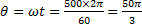# A motor is rotating at a constant angular velocity of 600 rpm. The angular displacement per second is a)b)c)d)## Question ID - 53733 :- A motor is rotating at a constant angular velocity of 600 rpm. The angular displacement per second is a)b)c)d)3537

 (d)rad
 A pulley of radius 2 m is rotating about its axis by a force=(20t -) N (whereis measured in seconds) applied tangentially. If the moment of inertia of the pulley about its axis rotation is 10, the number of rotations made by the pulley  before its direction of motion if reserved, is a) More than 3 but less than 6 b) More than 6 but less than 9 c) More than 9 d) Less than 3